|

# 陈益峰谈如何运用属相选择风水楼层?

现代住宅常常是多层的，甚至是高层建筑，在购买新房时候，看到那么多的楼层选择，很多购房者常常就懵头了。那么楼层的风水选择是否有讲究呢?如果有风水指导理论，那么应该如何考虑?小编专门请教了著名五行学家、风水学家陈益峰先生。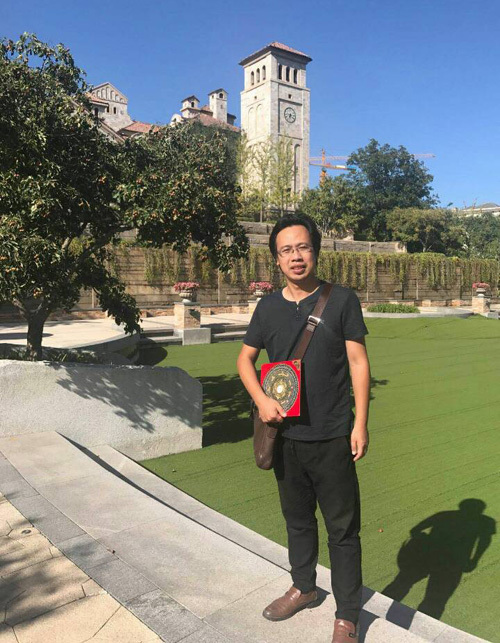陈益峰是著名风水五行学家，长期在国外传播与实践风水学说，在海内外风水行业具有崇高的威望和评价，先后前往澳大利亚、新加坡、文莱王国、马来西亚、德国、意大利、波兰等国家看风水，研究世界各地的阴阳五行的变化，总结了一套比较实用的风水心得。

陈先生是当代大陆第一个全面将中国的风水成功推向世界的风水学者，他擅长用专业英语，深入浅出的讲解阴阳五行理论，广泛的传播中国五行文化理论，得到国外广大风水爱好者的高度评价。陈师傅以实事求是的态度，以科学实用的精神，抛弃了中国风水行业一些糟粕的说法，探索出风水与人的真实规律关系，是风水届难得的一流高手。陈师傅认为楼层风水选择有一些讲究，当前专业上流行的做法大概有两种，一个是用神角度选择，一个是属相角度选择。陈师傅更加倾向于属相角度的选择。

陈师傅认为每一个属相有最适合自己的楼层，一般最好选择与自己的属相五行相生、相助的楼层，不要选择与自己属相相克、相泄的楼层。如果一个家庭的有夫妻两人，尽量考虑两人都适合的楼层，实在不行，就首先考虑房本主人的楼层。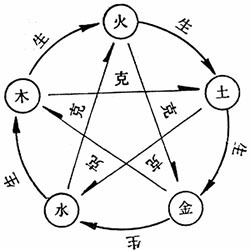1、五行

五行(the five elements)学说是风水的基础学说之一，风水的吉凶推断，都是依靠五行相生相克理论做出的。五行指木(wood)、火(fire)、土(soil)、金(meital)、水(water)，是构成宇宙的基本物质元素，在不同的事物上有不同的表现。其中行有相生（Inter-restriction）、相克（Inter-subjuation）的顺序。

五行相生的顺序是：木生火、火生土、土生金、金生水、水生木。

五行相克的顺序是：水克火、火克金、金克木、木克土、土克水。

木、火、土、金、水，五行与万物都有对应关系。比如：

（1）、对应的五色（color）：青、赤、黄、白、黑。

（2）、对应的五方(direction)：东、南、中、西、北。

（3）、对应的五季（season）：春、夏、长夏、秋、冬。

（4）、对应的五音(note)：角、徵[zhǐ]宫、商、羽。

（5）、对应的五脏：肝、心、脾、肺、肾。（the five internal organs (heart, liver, spleen, lungs and kidneys)）

（6）、对应的五腑（five hollow organs）：胆、小肠、胃、大肠、膀胱。（）

（7）、对应的五志（annals）：怒、喜、思、悲、恐。

（8）、对应的五官（the five sense organs）：目、舌、口、鼻、耳。

2、十二生肖

十二生肖，又叫属相（animal zodiac），是中国与十二地支相配以人出生年份的十二种动物，包括鼠（rat）、牛（ox）、虎（tiger）、兔（hare）、龙（dragon）、蛇（snake）、马（horse）、羊（sheep）、猴（monkey）、鸡（rooster）、狗（dog）、猪（boar）。十二生肖的起源与动物崇拜有关。原始社会的先民常用某种动物、无生物或自然现象的图形作为本氏族的保护神和标志，即图腾。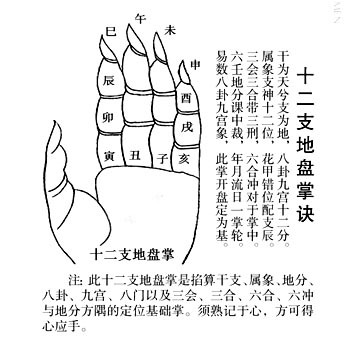3、十二地支

十二地支（the twelve earthly branches）分别为：子、丑、寅、卯、辰、巳、午、未、申、酉、戌、亥。

十二地支分为阴阳，其中阳支六个为：子、寅、辰、午、申、戌。

其中阴支六个为：丑、卯、巳、未、酉、亥。

4、地支与五行、方位

寅卯属木，寅阳木东北，卯阴木正东方；

巳午属火，午阳火南方，巳阴火东南方；

申酉属金，申阳金西南方，酉阴金正西方；

亥子属水，子阳水正北方，亥阴水西北方；

丑辰未戌属土，辰戌阳土，丑未阴土，丑东北方，辰东南方，未西南方，戌西北方。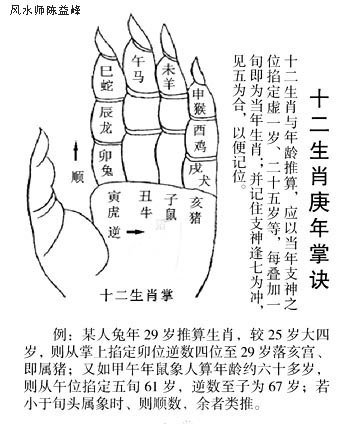5、十二支与生肖对应关系

子鼠、丑牛、寅虎、卯兔、辰龙、巳蛇、

午马、未羊、申猴、酉鸡、戍狗、亥猪。

6、数字(number)与河图（hetu）

河图中的十个数即为天地之数，故这十个数为先天之数。其中单数为阳，为天数;偶数为阴，为地数。河图口诀：一六共宗，二七同道，三八为朋，四九为友，五十同途。也就是北为水，一、六位于北;南为火，二、七位于南;东为木，三、八位于东;西为金，四、九位于西;中为土，五、十位于中。

所以一般的数字五行是：1、6属水，2、7属火，3、8属木，4、9属金，5、0属土。

7、出生年份与五行

每一个人出生的年份(year)对应一个干支(heavenly stems and earthly branches)，比如今年(2017年)出生的，干支为丁酉年，属相就是鸡。其他所有出生年份的干支可以查阅万年历得到。

甲子年、丙子年、戊子年、庚子年、壬子年，这些年份的生肖是属鼠，在五行方面属水。

乙丑年、丁丑年、已丑年、辛丑年、癸丑年，这些年份的生肖是属牛，在五行方面属土。

甲寅年、丙寅年、戊寅年、庚寅年、壬寅年，这些年份的生肖是属虎，在五行方面属木。

乙卯年、丁卯年、已卯年、辛卯年、癸卯年，这些年份的生肖是属兔，在五行方面属木。

甲辰年、丙辰年、戊辰年、庚辰年、壬辰年，这些年份的生肖是属龙，在五行方面属土。

乙巳年、丁巳年、已巳年、辛巳年、癸巳年，这些年份的生肖是属蛇，在五行方面属火。

甲午年、丙午年、戊午年、庚午年、壬午年，这些年份的生肖是属马，在五行方面属火。

乙未年、丁未年、已未年、辛未年、癸未年，这些年份的生肖是属羊，在五行方面属土。

甲申年、丙申年、戊申年、庚申年、壬申年，这些年份的生肖是属猴，在五行方面属金。

乙酉年、丁酉年、已酉年、辛酉年、癸酉年，这些年份的生肖是属鸡，在五生方面属金。

甲戌年、丙戌年、戊戌年、庚戌年、壬戌年，这些年份的生肖是属狗，在五行方面属土。

乙亥年、丁亥年、已亥年、辛亥年、癸亥年，这些年份的生肖是属猪，在五行方面属水。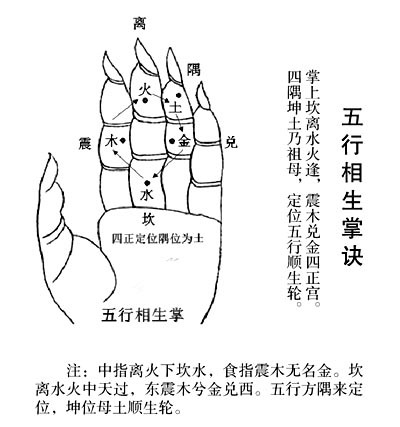8、楼层(floor)与五行

一楼和六楼属于北方，属水（water）。故楼房的第一层和第六层属水，尾数是一或六的层面，亦是属水，如十一楼、二十一楼、三十一楼等等。

二楼和七楼属于南方，属火(fire)。故楼房的第二层和第七层属火，尾数是二或七的层面，亦是属火，如十二楼、二十二楼、三十二楼等等。

三楼和八楼属于东方，属木(wood)。故楼房的第三层和第八层属木，尾数是三或八的层面，亦是属木，如十三楼，二十三楼、三十三楼等等。

四楼和九楼属于西方，属金(metal)。故楼房的第四层和第九层属金，尾数是四或九的层面，亦是属金，如十四楼、二十四楼、三十四楼等等。

五楼和十楼属于中央，属土(soil)。故楼房的第五层和第十层属土，尾数是五或十的层面，亦是属土，如十五楼、二十五楼、三十五楼等等。

五行的每一个元素都不是独立存在，而是互相依赖，互相制约的。其相生的次序是：火生土、土生金、金生水、水生木、木生火;相克的次序是火克金、金克木、木克土、土克水、水克火。9、属相与楼层

根据五行相生相克的原则，一般楼层的五行生或者助(五行相同为助)，为吉祥。楼层五行克或者泄属相的五行，为不吉祥。

属鼠的生肖吉利的(auspicious)楼层应该是一、四、六、九、十一、十四楼，不吉利的(Inauspicious)楼层有三、五、八、十、十三、十五楼。

属牛的生肖吉利的楼层应该是二、五、七、十、十二、十五楼，不吉利的楼层有三、四、八、九、十三、十四楼。

属虎的生肖吉利的楼层应该是一、三、六、八、十一、十三楼，不吉利的楼层有二、四、七、九、十二、十四楼。

属兔的生肖吉利的楼层应该是一、三、六、八、十一、十三楼，不吉利的楼层有二、四、七、九、十二、十四楼。

属龙的生肖吉利的楼层应该是二、五、七、十、十二、十五楼，不吉利的楼层有三、四、八、九、十三、十四楼。

属蛇的生肖吉利的楼层应该是二、三、七、八、十二、十三楼，不吉利的楼层有一、五、六、十、十一、十五楼。

属马的生肖吉利的楼层应该是二、三、七、八、十二、十三楼，不吉利的楼层有一、五、六、十、十一、十五楼。

属羊的生肖吉利的楼层应该是二、五、七、十、十二、十五楼，不吉利的楼层有三、四、八、九、十三、十四楼。

属猴的生肖吉利的楼层应该是四、五、九、十、十四、十五楼，不吉利的楼层有一、二、六、七、十一、十二楼。

属鸡的生肖吉利的楼层应该是四、五、九、十、十四、十五楼，不吉利的楼层有一、二、六、七、十一、十二楼。

属狗的生肖吉利的楼层应该是二、五、七、十、十二、十五楼，不吉利的楼层有三、四、八、九、十三、十四楼。

属猪的生肖吉利的楼层因该是一、四、六、九、十一、十四楼，不吉利的楼层有三、五、八、十、十三、十五楼。

楼层在风水选择中还是比较重要的，在实际运用中还需要活学活用，除了考虑属相的五行，还需要考虑室外的砂水（mountain or water）、凶煞（Demon wind）的情况。比如楼房住宅南面是一个高架桥，高度在8米左右。那么1、2、3、4楼层，无论是否符合宅主的五行，都不要选择。因为室外的高架桥属于煞气，煞气的作用力远大于楼层的五行作用力。（记者/黎明）

`声明：本文由入驻焦点开放平台的作者撰写，除焦点官方账号外，观点仅代表作者本人，不代表焦点立场错误信息举报电话： 400-099-0099，邮箱：jubao@vip.sohu.com，或点此进行意见反馈，或点此进行举报投诉。`A B C D E F G H J K L M N P Q R S T W X Y Z
A - B - C - D - E
• A
• 鞍山
• 安庆
• 安阳
• 安顺
• 安康
• 澳门
• B
• 北京
• 保定
• 包头
• 巴彦淖尔
• 本溪
• 蚌埠
• 亳州
• 滨州
• 北海
• 百色
• 巴中
• 毕节
• 保山
• 宝鸡
• 白银
• 巴州
• C
• 承德
• 沧州
• 长治
• 赤峰
• 朝阳
• 长春
• 常州
• 滁州
• 池州
• 长沙
• 常德
• 郴州
• 潮州
• 崇左
• 重庆
• 成都
• 楚雄
• 昌都
• 慈溪
• 常熟
• D
• 大同
• 大连
• 丹东
• 大庆
• 东营
• 德州
• 东莞
• 德阳
• 达州
• 大理
• 德宏
• 定西
• 儋州
• 东平
• E
• 鄂尔多斯
• 鄂州
• 恩施
F - G - H - I - J
• F
• 抚顺
• 阜新
• 阜阳
• 福州
• 抚州
• 佛山
• 防城港
• G
• 赣州
• 广州
• 桂林
• 贵港
• 广元
• 广安
• 贵阳
• 固原
• H
• 邯郸
• 衡水
• 呼和浩特
• 呼伦贝尔
• 葫芦岛
• 哈尔滨
• 黑河
• 淮安
• 杭州
• 湖州
• 合肥
• 淮南
• 淮北
• 黄山
• 菏泽
• 鹤壁
• 黄石
• 黄冈
• 衡阳
• 怀化
• 惠州
• 河源
• 贺州
• 河池
• 海口
• 红河
• 汉中
• 海东
• 怀来
• I
• J
• 晋中
• 锦州
• 吉林
• 鸡西
• 佳木斯
• 嘉兴
• 金华
• 景德镇
• 九江
• 吉安
• 济南
• 济宁
• 焦作
• 荆门
• 荆州
• 江门
• 揭阳
• 金昌
• 酒泉
• 嘉峪关
K - L - M - N - P
• K
• 开封
• 昆明
• 昆山
• L
• 廊坊
• 临汾
• 辽阳
• 连云港
• 丽水
• 六安
• 龙岩
• 莱芜
• 临沂
• 聊城
• 洛阳
• 漯河
• 娄底
• 柳州
• 来宾
• 泸州
• 乐山
• 六盘水
• 丽江
• 临沧
• 拉萨
• 林芝
• 兰州
• 陇南
• M
• 牡丹江
• 马鞍山
• 茂名
• 梅州
• 绵阳
• 眉山
• N
• 南京
• 南通
• 宁波
• 南平
• 宁德
• 南昌
• 南阳
• 南宁
• 内江
• 南充
• P
• 盘锦
• 莆田
• 平顶山
• 濮阳
• 攀枝花
• 普洱
• 平凉
Q - R - S - T - W
• Q
• 秦皇岛
• 齐齐哈尔
• 衢州
• 泉州
• 青岛
• 清远
• 钦州
• 黔南
• 曲靖
• 庆阳
• R
• 日照
• 日喀则
• S
• 石家庄
• 沈阳
• 双鸭山
• 绥化
• 上海
• 苏州
• 宿迁
• 绍兴
• 宿州
• 三明
• 上饶
• 三门峡
• 商丘
• 十堰
• 随州
• 邵阳
• 韶关
• 深圳
• 汕头
• 汕尾
• 三亚
• 三沙
• 遂宁
• 山南
• 商洛
• 石嘴山
• T
• 天津
• 唐山
• 太原
• 通辽
• 铁岭
• 泰州
• 台州
• 铜陵
• 泰安
• 铜仁
• 铜川
• 天水
• 天门
• W
• 乌海
• 乌兰察布
• 无锡
• 温州
• 芜湖
• 潍坊
• 威海
• 武汉
• 梧州
• 渭南
• 武威
• 吴忠
• 乌鲁木齐
X - Y - Z
• X
• 邢台
• 徐州
• 宣城
• 厦门
• 新乡
• 许昌
• 信阳
• 襄阳
• 孝感
• 咸宁
• 湘潭
• 湘西
• 西双版纳
• 西安
• 咸阳
• 西宁
• 仙桃
• 西昌
• Y
• 运城
• 营口
• 盐城
• 扬州
• 鹰潭
• 宜春
• 烟台
• 宜昌
• 岳阳
• 益阳
• 永州
• 阳江
• 云浮
• 玉林
• 宜宾
• 雅安
• 玉溪
• 延安
• 榆林
• 银川
• Z
• 张家口
• 镇江
• 舟山
• 漳州
• 淄博
• 枣庄
• 郑州
• 周口
• 驻马店
• 株洲
• 张家界
• 珠海
• 湛江
• 肇庆
• 中山
• 自贡
• 资阳
• 遵义
• 昭通
• 张掖
• 中卫

1室1厅1厨1卫1阳台

1
2
3
4
5

0
1
2

1

1

0
1
2
3报名成功，资料已提交审核A B C D E F G H J K L M N P Q R S T W X Y Z
A - B - C - D - E
• A
• 鞍山
• 安庆
• 安阳
• 安顺
• 安康
• 澳门
• B
• 北京
• 保定
• 包头
• 巴彦淖尔
• 本溪
• 蚌埠
• 亳州
• 滨州
• 北海
• 百色
• 巴中
• 毕节
• 保山
• 宝鸡
• 白银
• 巴州
• C
• 承德
• 沧州
• 长治
• 赤峰
• 朝阳
• 长春
• 常州
• 滁州
• 池州
• 长沙
• 常德
• 郴州
• 潮州
• 崇左
• 重庆
• 成都
• 楚雄
• 昌都
• 慈溪
• 常熟
• D
• 大同
• 大连
• 丹东
• 大庆
• 东营
• 德州
• 东莞
• 德阳
• 达州
• 大理
• 德宏
• 定西
• 儋州
• 东平
• E
• 鄂尔多斯
• 鄂州
• 恩施
F - G - H - I - J
• F
• 抚顺
• 阜新
• 阜阳
• 福州
• 抚州
• 佛山
• 防城港
• G
• 赣州
• 广州
• 桂林
• 贵港
• 广元
• 广安
• 贵阳
• 固原
• H
• 邯郸
• 衡水
• 呼和浩特
• 呼伦贝尔
• 葫芦岛
• 哈尔滨
• 黑河
• 淮安
• 杭州
• 湖州
• 合肥
• 淮南
• 淮北
• 黄山
• 菏泽
• 鹤壁
• 黄石
• 黄冈
• 衡阳
• 怀化
• 惠州
• 河源
• 贺州
• 河池
• 海口
• 红河
• 汉中
• 海东
• 怀来
• I
• J
• 晋中
• 锦州
• 吉林
• 鸡西
• 佳木斯
• 嘉兴
• 金华
• 景德镇
• 九江
• 吉安
• 济南
• 济宁
• 焦作
• 荆门
• 荆州
• 江门
• 揭阳
• 金昌
• 酒泉
• 嘉峪关
K - L - M - N - P
• K
• 开封
• 昆明
• 昆山
• L
• 廊坊
• 临汾
• 辽阳
• 连云港
• 丽水
• 六安
• 龙岩
• 莱芜
• 临沂
• 聊城
• 洛阳
• 漯河
• 娄底
• 柳州
• 来宾
• 泸州
• 乐山
• 六盘水
• 丽江
• 临沧
• 拉萨
• 林芝
• 兰州
• 陇南
• M
• 牡丹江
• 马鞍山
• 茂名
• 梅州
• 绵阳
• 眉山
• N
• 南京
• 南通
• 宁波
• 南平
• 宁德
• 南昌
• 南阳
• 南宁
• 内江
• 南充
• P
• 盘锦
• 莆田
• 平顶山
• 濮阳
• 攀枝花
• 普洱
• 平凉
Q - R - S - T - W
• Q
• 秦皇岛
• 齐齐哈尔
• 衢州
• 泉州
• 青岛
• 清远
• 钦州
• 黔南
• 曲靖
• 庆阳
• R
• 日照
• 日喀则
• S
• 石家庄
• 沈阳
• 双鸭山
• 绥化
• 上海
• 苏州
• 宿迁
• 绍兴
• 宿州
• 三明
• 上饶
• 三门峡
• 商丘
• 十堰
• 随州
• 邵阳
• 韶关
• 深圳
• 汕头
• 汕尾
• 三亚
• 三沙
• 遂宁
• 山南
• 商洛
• 石嘴山
• T
• 天津
• 唐山
• 太原
• 通辽
• 铁岭
• 泰州
• 台州
• 铜陵
• 泰安
• 铜仁
• 铜川
• 天水
• 天门
• W
• 乌海
• 乌兰察布
• 无锡
• 温州
• 芜湖
• 潍坊
• 威海
• 武汉
• 梧州
• 渭南
• 武威
• 吴忠
• 乌鲁木齐
X - Y - Z
• X
• 邢台
• 徐州
• 宣城
• 厦门
• 新乡
• 许昌
• 信阳
• 襄阳
• 孝感
• 咸宁
• 湘潭
• 湘西
• 西双版纳
• 西安
• 咸阳
• 西宁
• 仙桃
• 西昌
• Y
• 运城
• 营口
• 盐城
• 扬州
• 鹰潭
• 宜春
• 烟台
• 宜昌
• 岳阳
• 益阳
• 永州
• 阳江
• 云浮
• 玉林
• 宜宾
• 雅安
• 玉溪
• 延安
• 榆林
• 银川
• Z
• 张家口
• 镇江
• 舟山
• 漳州
• 淄博
• 枣庄
• 郑州
• 周口
• 驻马店
• 株洲
• 张家界
• 珠海
• 湛江
• 肇庆
• 中山
• 自贡
• 资阳
• 遵义
• 昭通
• 张掖
• 中卫• 手机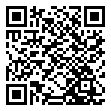• 分享
• 设计
免费设计
• 计算器
装修计算器
• 入驻
合作入驻
• 联系
联系我们
• 置顶
返回顶部